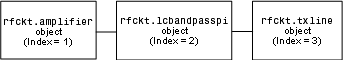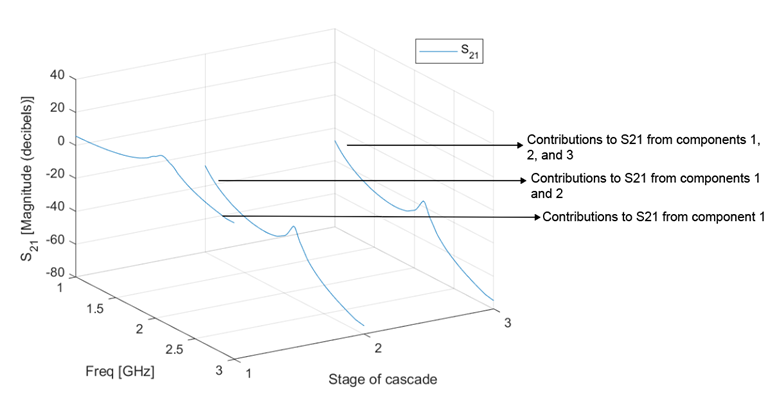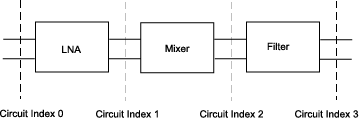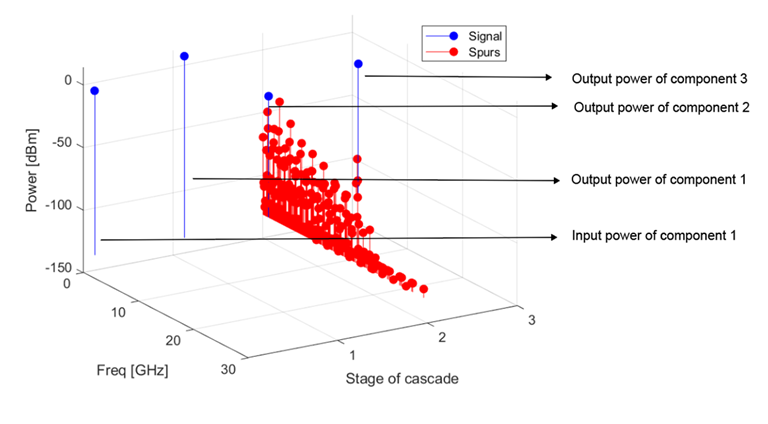## Analyze and Plot RF Components

### Analyze Networks in Frequency Domain

RF Toolbox™ lets you analyze RF components and networks in the frequency domain. You use the `analyze` function to analyze a circuit object over a specified set of frequencies.

For example, to analyze a coaxial transmission line from 1 GHz to 2.9 GHz in increments of 10 MHz:

```ckt = rfckt.coaxial; f = [1.0e9:1e7:2.9e9]; analyze(ckt,f); ```

Note

For all circuits objects except those that contain data from a file, you must perform a frequency-domain analysis with the `analyze` method before visualizing component and network data. For circuits that contain data from a file, the toolbox performs a frequency-domain analysis when you use the `read` method to import the data.

When you analyze a circuit object, the toolbox computes the circuit network parameters, noise figure values, and output third-order intercept point (OIP3) values at the specified frequencies and stores the result of the analysis in the object's `AnalyzedResult` property.

For more information, see the `analyze` function page.

### Visualize Component and Network Data

The RF Toolbox lets you validate the behavior of circuit objects that represent RF components and networks by plotting the following data:

• Large- and small-signal S-parameters

• Noise figure

• Output third-order intercept point

• Power data

• Phase noise

• Voltage standing-wave ratio

• Power gain

• Group delay

• Reflection coefficients

• Stability data

• Transfer function

This table summarizes the available plots and charts, along with the functions you can use to create each one and a description of its contents.

Plot Type

Functions

Plot Contents

Rectangular Plot

`plot`

`plotyy`

`loglog`

`semilogx`

`semilogy`

Parameters as a function of frequency or, where applicable, operating condition. The available parameters include:

• S-parameters

• Noise figure

• Voltage standing-wave ratio (VSWR)

• OIP3

Budget Plot

`plot`

Parameters as a function of frequency for each component in a cascade, where the curve for a given component represents the cumulative contribution of each RF component up to and including the parameter value of that component.

Mixer Spur Plot

`plot`

Mixer spur power as a function of frequency for an `rfckt.mixer` object or an `rfckt.cascade` object that contains a mixer.

Polar Plots and Smith Charts®

`polar`

`smithplot`

Polar plot: Magnitude and phase of S-parameters as a function of frequency.

Smith plot: Real and imaginary parts of S-parameters as a function of frequency, used for analyzing the reflections caused by impedance mismatch.

For each plot you create, you choose a parameter to plot and, optionally, a format in which to plot that parameter. The plot format defines how the RF Toolbox displays the data on the plot. The available formats vary with the data you select to plot. The data you can plot depends on the type of plot you create.

Note

You can use the `listparam` function to list the parameters of a specified circuit object that are available for plotting. You can use the `listformat` function to list the available formats for a specified circuit object parameter.

The following topics describe the available plots:

#### Rectangular Plot

You can plot any parameters that are relevant to your object on a rectangular plot. You can plot parameters as a function of frequency for any object. When you import object data from a `.p2d` or `.s2d` file, you can also plot parameters as a function of any operating condition from the file that has numeric values, such as bias. In addition, when you import object data from a `.p2d` file, you can plot large-signal S-parameters as a function of input power or as a function of frequency. These parameters are denoted `LS11`, `LS12`, `LS21`, and `LS22`.

This table summarizes the methods that are available in the toolbox for creating rectangular plots and describes the uses of each one. For more information on a particular type of plot, follow the link in the table to the documentation for that method.

MethodDescription
`plot`Plot of one or more object parameters
`plotyy`Plot of one or more object parameters with y-axes on both the left and right sides
`semilogx`Plot of one or more object parameters using a log scale for the X-axis
`semilogy`Plot of one or more object parameters using a log scale for the Y-axis
`loglog`Plot of one or more object parameters using a log-log scale

#### Budget Plot

You use the link budget or budget plot to understand the individual contribution of each component to a plotted parameter value in a cascaded network with multiple components. The budget plot shows one or more curves of parameter values as a function of frequency, ordered by the circuit index of the cascaded network.

```casc = rfckt.cascade('Ckts',... {rfckt.amplifier,rfckt.lcbandpasspi,rfckt.txline}) ```
This figure shows how the circuit index is assigned to each component in the cascade, based on its sequential position in the network.You create a 3-D budget plot for this cascade using the `plot` method with the second argument set to `'budget'`, as shown in the following command:

```analyze(casc,linspace(1e9,3e9,100)); plot(casc,'budget','s21') ```

Note that you have to `analyze` your circuit before plotting the budget plot and by default the budget plot is a 2-D plot. If you specify the array of frequencies in the `analyze` function you can visualize the budget results in 3-D. A curve on the budget plot for each circuit index represents the contributions to the parameter value of the RF components up to that index. This figure shows the budget plot.If you specify two or more parameters, the RF Toolbox puts the parameters in a single plot. You can only specify a single format for all the parameters.

#### Mixer Spur Plot

You use the mixer spur plot to understand how mixer nonlinearities affect output power at the desired mixer output frequency and at the intermodulation products that occur at the following frequencies:

`${f}_{out}=N\ast {f}_{in}+M\ast {f}_{LO}$`

where

• ${f}_{in}$ is the input frequency.

• ${f}_{LO}$ is the local oscillator frequency.

• N and M are integers.

The RF toolbox calculates the output power from the mixer intermodulation table (IMT). These tables are described in detail in the Visualize Mixer Spurs example.

The mixer spur plot shows power as a function of frequency for an `rfckt.mixer` object or an `rfckt.cascade` object that contains a mixer. By default, the plot is three-dimensional and shows a stem plot of power as a function of frequency, ordered by the circuit index of the object. You can create a two-dimensional stem plot of power as a function of frequency for a single circuit index by specifying the index in the mixer spur plot command.

```FirstCkt = rfckt.amplifier('NetworkData', ... rfdata.network('Type', 'S', 'Freq', 2.1e9, ... 'Data', [0,0;10,0]), 'NoiseData', 0, 'NonlinearData', inf); SecondCkt = read(rfckt.mixer, 'samplespur1.s2d'); ThirdCkt = rfckt.lcbandpasstee('L', [97.21 3.66 97.21]*1e-9, ... 'C', [1.63 43.25 1.63]*1.0e-12); CascadedCkt = rfckt.cascade('Ckts', ... {FirstCkt, SecondCkt, ThirdCkt}); ```
This shows how the circuit index is assigned to the components in the cascade, based on its sequential position in the network.• Circuit index 0 corresponds to the cascade input.

• Circuit index 1 corresponds to the LNA output.

• Circuit index 2 corresponds to the mixer output.

• Circuit index 3 corresponds to the filter output.

You create a spur plot for this cascade using the `plot` method with the second argument set to `'mixerspur'`, as shown in the following command:

```plot(CascadedCkt,'mixerspur') ```

Within the three dimensional plot, the stem plot for each circuit index represents the power at that circuit index. This figure shows the mixer spur plot.For more information on mixer spur plots, see the `plot` reference page.

#### Polar Plots and Smith Charts®

You can use the RF toolbox to generate Polar plots and Smith charts. If you specify two or more parameters, the RF toolbox puts the parameters in a single plot.

The following table describes the Polar plot and Smith charts options, as well as the available parameters.

Note

`LS11`, `LS12`, `LS21`, and `LS22` are large-signal S-parameters. You can plot these parameters as a function of input power or as a function of frequency.

Plot Type

Method

Parameter

`Polar plane`

`polar`

`S11`, `S12`, `S21`, `S22`

`LS11`, `LS12`, `LS21`, `LS22` (Objects with data from a P2D file only)

`Z Smith chart`

`smithplot` with `type` argument set to `'z'`

`S11`, `S22`

`LS11`, `LS22` (Objects with data from a P2D file only)

`Y Smith chart`

`smithplot` with `type` argument set to `'y'`

`S11`, `S22`

`LS11`, `LS22` (Objects with data from a P2D file only)

`ZY Smith chart`

`smithplot` with `type` argument set to `'zy'`

`S11`, `S22`

`LS11`, `LS22` (Objects with data from a P2D file only)

By default, the RF toolbox plots the parameter as a function of frequency. When you import block data from a `.p2d` or `.s2d` file, you can also plot parameters as a function of any operating condition from the file that has numeric values, such as bias.

Note

The `circle` method lets you place circles on a Smith® Chart to depict stability regions and display constant gain, noise figure, reflection and immittance circles. For more information about this function, see the `circle` reference page or Designing Matching Networks for Low Noise Amplifiers example about designing matching networks.

### Compute and Plot Time-Domain Specifications

The RF toolbox lets you compute and plot time-domain characteristics for RF components.

This section contains the following topics:

#### Compute Network Transfer Function

You use the `s2tf` function to convert 2-port S-parameters to a transfer function. The function returns a vector of transfer function values that represent the normalized voltage gain of a 2-port network.

The following code illustrates how to read a file data into a passive circuit object, extract the 2-port S-parameters from the object, and compute the transfer function of the data at the frequencies for which the data is specified. Here `z0` is the reference impedance of the S-parameters, `zs` is the source impedance, and `zl` is the load impedance. See the `s2tf` reference page for more information on how these impedances are used to define the gain.

```PassiveCkt = rfckt.passive('File','passive.s2p') z0=50; zs=50; zl=50; [SParams, Freq] = extract(PassiveCkt, 'S Parameters', z0); TransFunc = s2tf(SParams, z0, zs, zl); ```

#### Fit Model Object to Circuit Object Data

You use the `rationalfit` function to fit a rational function to the transfer function of a passive component. The `rationalfit` function returns an `rfmodel` object that represents the transfer function analytically.

The following code illustrates how to use the `rationalfit` function to create an `rfmodel.rational` object that contains a rational function model of the transfer function that you created in the previous example.

`RationalFunc = rationalfit(Freq, TransFunc)`

To find out how many poles the RF toolbox used to represent the data, look at the length of the `A` vector of the `RationalFunc` model object.

```nPoles = length(RationalFunc.A) ```

Note

The number of poles is important if you plan to use the RF model object to create a model for use in another simulator, because a large number of poles can increase simulation time. For information on how to represent a component accurately using a minimum number of poles, see Represent Circuit Object with Model Object.

Use the `freqresp` function to compute the frequency response of the fitted data. To validate the model fit, plot the transfer function of the original data and the frequency response of the fitted data.

```Resp = freqresp(RationalFunc, Freq); plot(Freq, 20*log10(abs(TransFunc)), 'r', ... Freq, 20*log10(abs(Resp)), 'b--'); ylabel('Magnitude of H(s) (decibels)'); xlabel('Frequency (Hz)'); legend('Original', 'Fitting result'); title(['Rational fitting with ', int2str(nPoles), ' poles']); ```

#### Compute and Plot Time-Domain Response

You use the `timeresp` function to compute the time-domain response of the transfer function that `RationalFunc` represents. This code illustrates how to create a random input signal, compute the time-domain response of `RationalFunc` to the input signal, and plot the results.

```SampleTime=1e-11; NumberOfSamples=4750; OverSamplingFactor = 25; InputTime = double((1:NumberOfSamples)')*SampleTime; InputSignal = ... sign(randn(1, ceil(NumberOfSamples/OverSamplingFactor))); InputSignal = repmat(InputSignal, [OverSamplingFactor, 1]); InputSignal = InputSignal(:); [tresp,t]=timeresp(RationalFunc,InputSignal,SampleTime); plot(t*1e9,tresp); title('Fitting Time-Domain Response', 'fonts', 12); ylabel('Response to Random Input Signal'); xlabel('Time (ns)');```

For more information about computing the time response of a model object, see the `timeresp` function.